## Main

The first realization of quantum dense coding was optical, using pairs of photons entangled in polarization2. Dense coding has since been realized in various physical systems and broadened theoretically to include high-dimension quantum states with multiparties11, and even coding of quantum states12. The protocol extension to continuous variables13,14 has also been experimentally explored optically, using superimposed squeezed beams15. Other physical approaches include a simulation in nuclear magnetic resonance with temporal averaging16, and an implementation with atomic qubits on demand without postselection17. However, photons remain the optimal carriers of information given their resilience to decoherence and ease of creation and transportation.

Quantum dense coding was conceived1 such that Bob could communicate 2 bits of classical information to Alice with the transmission of a single qubit, as follows. Initially, each party holds one spin-1/2 particle of a maximally entangled pair, such as one of the four Bell states. Bob then encodes his 2-bit message by applying one of four unitary operations on his particle, which he then transmits to Alice. Finally, Alice decodes the 2-bit message by discriminating the Bell state of the pair.

Alice’s decoding step, deterministically resolving the four Bell states, is known as Bell-state analysis (BSA). Although in principle attainable with nonlinear interactions, such BSA with photons is very difficult to achieve with present technology, yielding extremely low efficiencies and low discrimination fidelities18. Therefore, current fundamental studies and technological developments demand the use of linear optics. However, for quantum communication, standard BSA with linear optics is fundamentally impossible3,4. At best, only two Bell states can be discriminated; for quantum communication, the other two are considered together for a three-message encoding. Consequently, the maximum channel capacity of this conventional optical dense coding is log23≈1.585 bits. Although there are probabilistic approaches that can distinguish all four Bell states (which would be necessary to achieve the fundamental channel capacity of 2), these are at best successful 50% of the time19, so have a net channel capacity of at most 1 per photon.

Entanglement in an extra degree of freedom (DOF) of the pair, hyperentanglement20, enables full BSA with linear optics5,6. In this case, because Bob only encodes information in one DOF (the auxiliary DOF is unchanged), a dense-coding protocol proceeds under the same encoding conditions as in the original proposal1. Although hyperentanglement-assisted BSA (HBSA) on polarization states has been reported with ancillas entangled in energy–time6 and linear momentum7, no advantage for quantum information or fundamental physics was shown; experiments thus far have been limited to a channel capacity of less than 1.18(3) bits6, substantially less than is possible even without hyperentangled resources.

Using pairs of photons entangled in their spin and orbital angular momentum (OAM) in a HBSA with high stability and high detection fidelity, we realize a dense-coding experiment with a channel capacity that exceeds the threshold to beat conventional linear-optics schemes. In our scheme, Alice and Bob are provided with pairs of photons simultaneously entangled in their spin and ±1-OAM in a state of the formHere, H (V) represents the horizontal (vertical) photon polarization and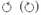represents the paraxial spatial modes (Laguerre–Gauss) carrying + (−) units of OAM21. Bob encodes his message by applying one of four unitary operations on the spin of his photon of this hyperentangled pair: (1) the identity, (2) V →−V, (3)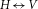or (4) V →−V and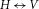. Such operations transform the state in equation (1) into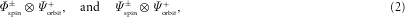where the spin and orbit Bell states are defined as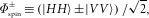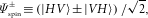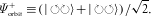We designed a HBSA scheme (inspired by ref. 22) enabling Alice to discriminate the four states in equation (2). In this scheme, the polarization BSA relies on the observation that the states resulting from Bob’s encoding can be rewritten as superpositions of the single-photon Bell states of spin and OAM, or spin–orbit Bell states: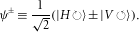On this basis, the states Alice analyses have the form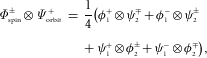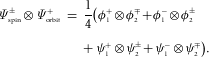This arrangement shows that each hyperentangled state is a unique superposition of four of the sixteen possible combinations of two-photon spin–orbit Bell states. Therefore, Alice can decode Bob’s message by carrying out spin–orbit BSA locally on each photon.

We implement the spin–orbit BSA with a novel interferometric apparatus consisting of a ±1-OAM splitter and polarizing beam splitters (PBSs), as shown in Fig. 1. The first splitter combines the action of a binary plane-wave phase grating21 and single-mode fibres. The grating transforms an incoming photon in the state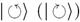into a gaussian beam with no OAM in the +1 (−1) diffraction order (for a splitter that preserves the photon’s OAM, see ref. 21). Subsequently filtering the first diffraction orders with single-mode fibres, we effectively split an incoming photon into its ±1-OAM components. By merging these diffraction orders on a PBS, we carry out a spin-controlled NOT gate over the photon OAM. In Fig. 1, the states ψ± (φ±) exit on the top (bottom) output port of the PBS. Followed by measurements in the diagonal basis, shown in Fig. 1 as PBS@45, the desired measurement in the single-photon Bell-state basis is accomplished. Further birefringent elements make this device a universal unitary gate for single-photon two-qubit states, in analogy with the device for polarization–linear momentum states in ref. 23.

Each step in the dense-coding protocol corresponds to a distinct experimental stage in Fig. 2: a hyperentanglement source, Bob’s encoding components and Alice’s HBSA. The hyperentanglement source is realized via spontaneous parametric downconversion in a pair of nonlinear crystals (see the Methods section). The generated photon pairs are entangled in polarization, OAM and emission time9. In particular, we use a subspace of the produced states that was shown to have a state overlap or fidelity of 97% with the state in equation (1). Next, Bob encodes his message in the polarization state by applying birefringent phase shifts with a pair of liquid crystals, as shown in Fig. 2. Finally, Alice carries out HBSA using two of the spin–orbit Bell-state analysers shown in Fig. 1, one for each photon (see the Methods section).Figure 2: Experimental set-up for dense coding with spin–orbit encoded photons.

We characterize our dense-coding implementation by switching between the four states for equal intervals, and measuring all output states of the HBSA. The results of these measurements are coincidence counts for each input state, as shown in Fig. 3. From this data, we can determine the conditional detection probabilities that Alice detects each message Φ± and Ψ± that Bob sent, for example, the message Φ+. The probabilities shown in Fig. 4 were calculated by comparing the sum of the four rates corresponding to each detected message over the sum of all sixteen rates for the sent message. The average probability of success was 94.8(2)% (all reported errors from Monte Carlo simulations).Figure 4: Conditional detection probabilities beating the channel capacity limit for standard dense coding with linear optics.

A better figure of merit for a quantum dense-coding implementation is the channel capacity, because it characterizes the exponential growth of the maximum number of distinguishable signals for a given number of uses of the channel (see the Methods section). From the conditional detection probabilities, we obtain a channel capacity of 1.630(6) bits with a probability of sending each state of P(Φ+)=0.26, P(Φ)=0.26, P(Ψ+)=0.24 and P(Ψ)=0.24. This exceeds the 1.585 channel capacity threshold for conventional linear-optics implementations. The channel capacity drifted by no more than one standard deviation between experimental runs, demonstrating the high stability of the implementation.

The experimental channel capacity is nevertheless smaller than the maximum attainable (2 bits), owing to imperfections in the alignment, input states and components. By characterizing each imperfection and modelling the gates and measurement, we estimated their effect on the channel capacity (see Supplementary Information, Part II). Considering all mentioned imperfections (see the Methods section) and their spread in a Monte Carlo simulation, the predicted channel capacity of 1.64(2) bits agrees with the measured channel capacity of 1.630(6) bits. The polarization and spatial-mode states can be improved by spatially compensating the angle-dependent phase24, using a forked hologram with a smaller diffraction angle to decrease wavelength dispersion (a potential source of alignment imbalances), and obtaining crystals with a smaller wedge. The deleterious effect of the PBS crosstalk can be reduced by adding extra phase-compensation plates inside the interferometers, and can potentially be eliminated altogether by adding appropriate birefringent beam displacers after each PBS.

Above, Bob encoded two qubits in the form of spin–orbit Bell states by acting only on the spin DOF. However, more generally he could also apply one of four unitaries in the ±1-OAM subspace and encode four qubits. The state of the pair of photons then becomes a product of Bell states, 16 in total. In principle, if Alice could discriminate all of these ‘hyper-Bell’ states, up to 4 bits could be transmitted per photon. We have investigated the limits for unambiguously distinguishing these Bell-like states, and have found that the optimal one-shot discrimination scheme is to group the 16 states into 7 distinguishable classes25. The optimal analysis can be achieved by the Kwiat–Weinfurter scheme5, with photon-number resolving detectors, giving a maximum channel capacity of log27≈2.81 bits. If we modify the present scheme, we can also implement an unambiguous discrimination of all 16 Bell states with two identical copies25.

In conclusion, we have beaten a fundamental limit on the channel capacity for standard dense coding using only linear optics. A number of features make our HBSA efficient and reliable. First, hyperentanglement offers advantages in the source, logic gates and detection side. Quantum logic between qubits encoded on different DOFs is much more easily implemented than when using different photons26,27. From the source side, more quantum information is available per photon, particularly with the energy–time and spatial-mode DOF (for example, ref. 28). In the detection side, compared with multiphoton approaches, higher efficiency is achieved because only one pair of photons is detected. Second, because our HBSA requires only local measurements, Alice can measure one of the photons and store the classical result of her measurement until Bob sends his photon (she does not require a quantum memory). Finally, the photon’s polarization and ±1-OAM constitute a robust encoding as they enable quantum communication without alignment10 as well as other landmark advances for quantum information8. Furthermore, by using paraxial beams as the ancillary DOF, the scheme is free of tight source-to-detector requirements such as interferometric stability7 or perfect indistiguishability for Hong–Ou–Mandel interference6. However, OAM single-photon and entangled states easily decohere by atmospheric turbulence29,30, limiting their likely communication applications to satellite-to-satellite transmissions.

## Methods

The hyperentanglement source is realized by directing 120 mW of 351 nm light from a continuous-wave Ar+ laser into two contiguous β-barium borate nonlinear crystals with optic axes aligned in perpendicular planes9. Type-I degenerate 702 nm photons in a 3.0 half-opening angle cone are produced by phase-matching each 0.6-mm-thick crystal. In the spin and ±1 OAM subspace, a two-fold coincidence rate of five detected pairs per second is determined by a 10 ns coincidence window and interference filters with ΔλFWHM=5 nm.

In our HBSA implementation, each PBS@45 and its two outputs in the spin–orbit BSA (Fig. 1) were replaced by a dichroic polarizer oriented at either 45 or −45 and a single output; Alice’s HBSA thus acquires all spin–orbit BSA outputs from four polarizer settings. With the continuous-wave source, Alice cycles through the four polarizer settings, and for each polarizer setting Bob encodes the four messages, each for 150 s. During the measurement, no active stabilization or realignment was done on the source, spin–orbit BSA interferometers or coupling optics. The HBSA polarizers and liquid crystals were quickly set with computer-controlled rotation stages and liquid-crystal controllers.

The wavelength-dependent voltage applied to each liquid crystal was independently calibrated to produce a birefringent phase difference of 0 or π with a diode laser operated at 699 nm (Hitachi HL-6738MG, driven at 140 mA and 80 C); the same laser was used to align the ±1-OAM splitter. The binary forked holograms were silver-halide emulsion gratings with 33% diffraction efficiency into the first order (more efficient schemes are described in ref. 21). The same holographic plate included spatial-mode tomography patterns, which in conjunction with the liquid crystals were used for state reconstruction9. The spurious phase on reflection on the PBS was compensated with a waveplate in each output port of the PBS for both spin–orbit Bell-state analysers. The state-discrimination signal-to-noise ratio (SNR) varied between states owing to mode-coupling imbalance in the spin–orbit BSA, PBS crosstalk and slight offsets in the liquid crystal calibrations.

We characterized the source polarization state Φspin by quantum state tomography in theOAM subspaces9 (using liquid crystals shown in Fig. 2 and PBSs of each spin–orbit BSA shown in Fig. 1). Considering all combinations of signature detectors, we measured an average degree of entanglement, or tangle, of T=96.7(8)% and a mixture or linear entropy of SL=2.0(4)%. If such high-quality polarization states were exactly the same for each combination of signature detectors, the decrease in the channel capacity would be only 0.006 bits. However, small differences in the coupled state between each combination of detectors (expressed above as uncertainty) result in a channel capacity decrease of 0.09(2) (see Supplementary Information, Part II). The OAM state was also tomographically reconstructed in the |H H〉 and |V V 〉 polarization subspaces9, measuring an average T=91(3)% and SL=6(2)%, yielding a channel capacity decrease of 0.20(3) bits. The PBS crosstalk (0.5% for H, 1.0% for V) further decreases the channel capacity by 0.10(1) bits. Finally, accidental coincidences (5 in 150 s) reduce channel capacity by 0.02 bits.

### Channel capacity

The capacity of a noisy channel is given by CC=maxp(x)H(X:Y), where x is in the space of signals that can be transmitted X, H(X:Y) is the mutual information of X and the space of received signals Y and the maximum is taken over all input distributions p(x). H(X:Y) is a function of p(x) and the conditional detection distribution p(y|x) of receiving y given that x was sent: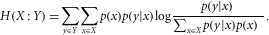In our experiment, a uniform probability of transmission gives a mutual information of 1.629(6) bits, negligibly smaller than the channel capacity owing to the nearly balanced conditional probabilities, that is, there is little to be gained by sending some states more frequently.